# Question(s) from Search: IIT

Search Results Difficulty Solution
1

The equation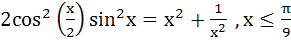has

a) No solution

b) One solution

c) More than one real solution

d) Cannot be said

The equationhas

a) No solution

b) One solution

c) More than one real solution

d) Cannot be said

IIT 1980
01:57 min
2

The number of solutions of the equation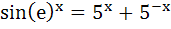a) 0

b) 1

c) 2

d) Infinitely many

The number of solutions of the equationa) 0

b) 1

c) 2

d) Infinitely many

IIT 1990
01:46 min
3

The number of values of x in the interval (0, 5π) satisfying the equation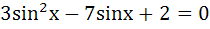is

a) 0

b) 5

c) 6

d) 10

The number of values of x in the interval (0, 5π) satisfying the equationis

a) 0

b) 5

c) 6

d) 10

IIT 1998
03:17 min
4

Find the natural number a for which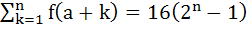where the function f satisfies the relation f (x + y) = f (x).f(y)for all natural numbers x and y and further f (1) = 2

Find the natural number a for whichwhere the function f satisfies the relation f (x + y) = f (x).f(y)for all natural numbers x and y and further f (1) = 2

IIT 1992
06:01 min
5

If α + β =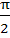and β + γ = α, then tanα equals

a) 2(tanβ + tanγ)

b) tanβ + tanγ

c) tanβ + 2tanγ

d) 2tanβ + tanγ

If α + β =and β + γ = α, then tanα equals

a) 2(tanβ + tanγ)

b) tanβ + tanγ

c) tanβ + 2tanγ

d) 2tanβ + tanγ

IIT 2001
02:03 min
6

Let n be a positive integer and
(1 + x + x2)n = a0 + a1x + a2x + a2x2 +  .  .  . + a2nx2n then prove that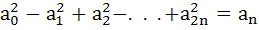Let n be a positive integer and
(1 + x + x2)n = a0 + a1x + a2x + a2x2 +  .  .  . + a2nx2n then prove thatIIT 1994
06:48 min
7

The larger of 9950 + 10050 and 10150  is

The larger of 9950 + 10050 and 10150  is

IIT 1982
04:38 min
8

Find all solutions of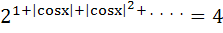in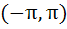a)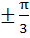b)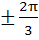c)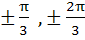d)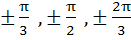Find all solutions ofina)b)c)d)IIT 1984
03:20 min
9

Let f (x) = sin x and g (x) = ln|x|. If the range of the composition

functions fog and gof are R1 and R2 respectively, then

a) R1 = [ u : −1 ≤ u < 1], R2 = [ v : −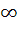< v < 0 ]

b) R1 = [ u : −< u < 0 ], R2 = [ v : −1 ≤ v ≤ 0]

c) R1 = [ u : −1 < u < 1], R2 = [ v : −< v < 0 ]

d) R1 = [ u : −1 ≤ u ≤ 1], R2 = [ v : −< v ≤ 0 ]

Let f (x) = sin x and g (x) = ln|x|. If the range of the composition

functions fog and gof are R1 and R2 respectively, then

a) R1 = [ u : −1 ≤ u < 1], R2 = [ v : −< v < 0 ]

b) R1 = [ u : −< u < 0 ], R2 = [ v : −1 ≤ v ≤ 0]

c) R1 = [ u : −1 < u < 1], R2 = [ v : −< v < 0 ]

d) R1 = [ u : −1 ≤ u ≤ 1], R2 = [ v : −< v ≤ 0 ]

IIT 1994
03:03 min
10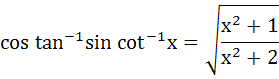a) True

b) Falsea) True

b) False

IIT 2002
02:39 min
11

Multiple choices
y = f ( x ) =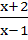then

a) x = f (y)

b) f (1) = 3

c) y is increasing with x for x < 1

d) f is a rational function of x

Multiple choices
y = f ( x ) =then

a) x = f (y)

b) f (1) = 3

c) y is increasing with x for x < 1

d) f is a rational function of x

IIT 1989
01:29 min
12

Let f (x + y) = f (x) f (y) for all x, y. Suppose that f (5) = 2 and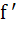(0) = 3. Find f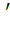(5).

a) 1

b) 2

c) 3

d) 6

Let f (x + y) = f (x) f (y) for all x, y. Suppose that f (5) = 2 and(0) = 3. Find f(5).

a) 1

b) 2

c) 3

d) 6

IIT 1981
03:33 min
13

In a triangle PQR, sin P, sin Q, sin R are in arithmetic progression then

a) Altitudes are in arithmetic progression

b) Altitudes are in harmonic progression

c) Medians are in geometric progression

d) Medians are in arithmetic progression

In a triangle PQR, sin P, sin Q, sin R are in arithmetic progression then

a) Altitudes are in arithmetic progression

b) Altitudes are in harmonic progression

c) Medians are in geometric progression

d) Medians are in arithmetic progression

IIT 1998
03:36 min
14

The external radii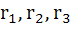of ΔABC are in harmonic progression then prove that a, b, c are in arithmetic progression

a) True

b) False

The external radiiof ΔABC are in harmonic progression then prove that a, b, c are in arithmetic progression

a) True

b) False

IIT 1983
01:51 min
15

True / False

If f (x) = ( a – xn )1/n  where a > 0 and n is a positive integer then f ( f ( x ) ) = x.

a) True

b) False

True / False

If f (x) = ( a – xn )1/n  where a > 0 and n is a positive integer then f ( f ( x ) ) = x.

a) True

b) False

IIT 1983
01:23 min
16

Fill in the blank

The domain of the function f (x) =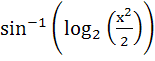is

a) [− 2, − 1]

b) [1, 2]

c) [− 2, − 1] ⋃ [1, 2]

d) None of the above

Fill in the blank

The domain of the function f (x) =is

a) [− 2, − 1]

b) [1, 2]

c) [− 2, − 1] ⋃ [1, 2]

d) None of the above

IIT 1984
02:48 min
17

Both roots of the equation

( x – b) ( x – c) + (x – c) ( x – a) + (x – a) (x – b) = 0 are always

a) positive

b) negative

c) real

d) none of these

Both roots of the equation

( x – b) ( x – c) + (x – c) ( x – a) + (x – a) (x – b) = 0 are always

a) positive

b) negative

c) real

d) none of these

IIT 1980
02:52 min
18

Two towns A and B are 60 meters apart. A school is to be built to serve 150 students in town A and 50 students in town B. If the total distance to be travelled by all the 200 students is to be as small as possible then the school should be built at

a) Town B

b) 45 km from town A

c) Town A

d) 45 km from town B

Two towns A and B are 60 meters apart. A school is to be built to serve 150 students in town A and 50 students in town B. If the total distance to be travelled by all the 200 students is to be as small as possible then the school should be built at

a) Town B

b) 45 km from town A

c) Town A

d) 45 km from town B

IIT 1982
01:37 min
19

If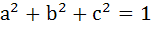then ab + bc + ca lies in the interval

a)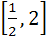b)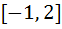c)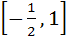d)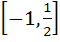Ifthen ab + bc + ca lies in the interval

a)b)c)d)IIT 1984
02:29 min
20

Let α, β be roots of the equation (x – a) (x – b) = c, c ≠ 0. Then the roots of the equation (x – α) (x – β) + c = 0 are

a) a, c

b) b, c

c) a, b

d) a + c, b + c

Let α, β be roots of the equation (x – a) (x – b) = c, c ≠ 0. Then the roots of the equation (x – α) (x – β) + c = 0 are

a) a, c

b) b, c

c) a, b

d) a + c, b + c

IIT 1992
02:15 min
21

If p, q ε {1, 2, 3, 4}. The number of equations of the form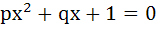having real roots is

a) 15

b) 9

c) 7

d) 8

If p, q ε {1, 2, 3, 4}. The number of equations of the formhaving real roots is

a) 15

b) 9

c) 7

d) 8

IIT 1994
03:39 min
22

For all x ε ( 0, 1 )

a)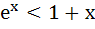b) ln (1 + x) < x

c) sinx > x

d) lnx > x

For all x ε ( 0, 1 )

a)b) ln (1 + x) < x

c) sinx > x

d) lnx > x

IIT 2000
02:40 min
23

The number of values of k for which the system of equations

(k + 1) x + 8y = 4k

kx + ( k + 3 ) y = 3k – 1

has infinitely many solutions is

a) 0

b) 1

c) 2

d) Infinity

The number of values of k for which the system of equations

(k + 1) x + 8y = 4k

kx + ( k + 3 ) y = 3k – 1

has infinitely many solutions is

a) 0

b) 1

c) 2

d) Infinity

IIT 2002
02:56 min
24

If f (x) =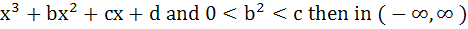a) f (x) is a strictly increasing function

b) f (x) has a local maxima

c) f (x) is a strictly decreasing function

d) f (x) is bounded

If f (x) =a) f (x) is a strictly increasing function

b) f (x) has a local maxima

c) f (x) is a strictly decreasing function

d) f (x) is bounded

IIT 2004
02:07 min
25

Let Δa =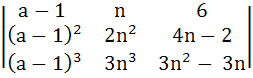Then show that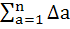= c, a constant.

Let Δa =Then show that= c, a constant.

IIT 1989
05:34 min

Play Selected  Login to save this search...